## New data

I ran the Minkowski ansatz for all 4319 3-dimensional reflexive polytopes, calculating the first 100 power series coefficients of the principal period for each of them.  The results were as follows.

3025 of the polytopes had a non-empty list of Minkowski polynomials, and 670 of them had more than one Minkowksi polynomial.

There were 165 distinct sequences of power series coefficients.  I then looked for differential operators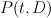$P(t,D)$ annilihating the principal period such that$P(t,D)$ has degree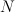$N$ in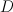$D$ and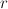$r$ in$t$, where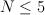$N \leq 5$ and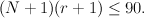$(N+1)(r+1)\leq 90.$  The results were:

N=3:	23 examples in total

r=2:	3 examples
r=3:	2 examples
r=4:	18 examples

N=4:	49 examples in total

r=7:	5 examples
r=8:	3 examples
r=9:	14 examples
r=10:	27 examples

N=5:	10 examples in total

r=11:	1 example
r=12:	2 examples
r=13:	0 examples
r=14:	7 examples

So in particular we found 23 D3 forms.

1.Sergei says:

Tom, could you please sieve the equations (classes of polytopes with the same equation) into the following excluding categories:

1. [18 cases] Classes with a smooth toric Fano representative
2. [+44 cases] Classes with conifold (terminal Gorenstein Fano) representative
3. [3-5 cases] Equations for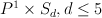$P^1 \times S_d, d \leq 5$
4. The rest classes/equations

1. Classes with a smooth polytope representative falls under [Givental’s theorem]:
The corresponding Fano is this representative itself.

2. Classes with a terminal Gorenstein polytope representative fall under
[Batyev’s toric degeneration ansatz/hypothesis]:
The corresponding Fano is the smoothing, it exists and can be determined by
http://member.ipmu.jp/sergey.galkin/3a.pdf (pp 11-13).
Invariants $\rho$ and $d(X)$ can be computed by
this GP script (it’s input is the coordinates of the vertices for the polytope and it’s dual).

3. Equations for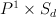$P^1 \times S_d$

Smooth Fano varieties fall into the dichotomy:
either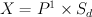$X = P^1 \times S_d$, where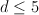$d \leq 5$
or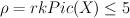$\rho = rk Pic (X) \leq 5$

Moreover, in the case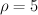$\rho=5$ there are 3 families: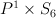$P^1 \times S_6$ of degree 36, one other toric variety of degree 36 and one nontoric variety of degree 28 (and it admits no degenerations to terminal Gorenstein toric varieties).

Del Pezzo surfaces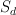$S_d$ for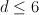$d \leq 6$ are G-Fanos.
Blowup of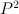$P^2$ in 1 or 2 points has G-invariant Picard number equal to 2.
Hence the G-invariant Picard of their product with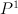$P^1$ is respectively 2 or 3.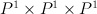$P^1 \times P^1 \times P^1$ turns out to be G-Fano itself.

So except for (possible) two cases of Fano varieties with$\rho=5$
all other smooth Fano threefolds have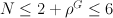$N \leq 2 + \rho^G \leq 6$.

Since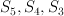$S_5, S_4, S_3$ have Gorenstein degenerations, multiplied by$P^1$ they also have Gorenstein degenerations. I am not sure whether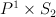$P^1 \times S_2$ and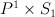$P^1 \times S_1$ have Gorenstein degenerations.$P^1 \times S_1$ has non-free anticanonical system so probably it haven’t Gorenstein degenerations: anticanonical systems of Gorenstein Fanos are free, however the limit of the base locus for smooth threefolds may move along the singularity curve of the singular depending on the degeneration.

Number r looks suspiciously big.
What is r in the case of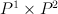$P^1 \times P^2$?

2.Sergei says:

Also, please check for the equations with N=6.

Numbers of families with the given rank of Picard group are the following
(I excluded 5 Fanos with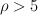$\rho>5$)

 |------------------------------------------ | 1| 2| 3| 4|5| < === $\rho$ |------------------------------------------ |17|36|31|13|3| <=== 100 smooth Fanos |------------------------------------------ | 1| 4| 7| 4|2| <=== 18 smooth toric | 4|16|16| 8|0| <=== 44 with STD |------------------------------------------ |12|16| 8| 1|1| <=== The rest ------------------------------------------- 

For 10 out of 12 remaining Fanos with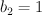$b_2=1$ there is a Gorenstein degeneration.
For other 2 probably there are no Gorenstein degeneration. In case of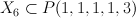$X_6 \subset P(1,1,1,1,3)$ it's easy to prove - dual Gorenstein polytope should have volume 2 which is impossible.
In case of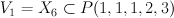$V_1 = X_6 \subset P(1,1,1,2,3)$ maybe one should con sider the 1/2-homothety of the dual polytope).

So only a quarter of the families are left for the consideration.
Specifying some of the conventional invariants could help to furnish it further. Degree is the same for smooth and Gorenstein varieties. Upper bound of Gorenstein Picard numbers gives a lower bound for smooth Picard number.

3.Sergei says:

Another question that bother me is the following:
there are 100 terminal Gorenstein polytopes and
100 of their duals (mostly non-terminal).

What Minkowski ansatz produces for these duals?